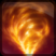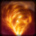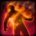# Feu du dragonConditions

## Related effects, buffs and debuffs

Please click on an effect below to view its details.

• [does not expire]Souffle du dragon
Dégâts élémentaires chaque seconde.

 Slot: Buff Duration: passive Tick rate: 1s # occurrences: 1 Target overrides: [target override] Aoe Sphere
• When effect ticks
• [target override]

Perform the following actions:

• Add effect #2 to TARGET from TARGET
• When channel finished

Perform the following actions:

• Play appearance epp . npc . ability . skytrooper . firestorm_channel_exit, dependent on calling effect
• When effect is applied

Perform the following actions:

• Immobilize and prevent rotation
• [3s]Brûlure
Dégâts élémentaires réguliers.

 Slot: Debuff Duration: 3s Tick rate: 1s Initial stacks: 1 Max stacks: 3 # occurrences: 1 Tags: tag.​abl.​debuff.​damage_over_time, tag.​abl.​debuff.​burn Conditions:
• On Apply

Perform the following actions:

• Play appearance epp . npc . ability . skytrooper . firestorm_burn_target, dependent on calling effect
• When effect ticks

Only when the following conditions are met:

• If stacks of this effect = 1

Perform the following actions:

• Spell Damage
- Unknown (609) = (bool) false
- Damage Type = (int) 3
- Slot = (int) 1
- Spell Type = (int) 2
- Level Cap = (int) 0
- Amount Max = (float) 0
- Amount Min = (float) 0
- Amount Percent = (float) 0
- Threat Percent = (float) 0
- Standard Health Percent Max = (float) 0.05
- Standard Health Percent Min = (float) 0.04
- Amount Modifier Percent = (float) 0
- Coefficient = (float) 0
- Health Steal Percentage = (float) 0
• When effect ticks

Only when the following conditions are met:

• If stacks of this effect = 2

Perform the following actions:

• Spell Damage
- Unknown (609) = (bool) false
- Damage Type = (int) 3
- Slot = (int) 1
- Spell Type = (int) 2
- Level Cap = (int) 0
- Amount Max = (float) 0
- Amount Min = (float) 0
- Amount Percent = (float) 0
- Threat Percent = (float) 0
- Standard Health Percent Max = (float) 0.07
- Standard Health Percent Min = (float) 0.06
- Amount Modifier Percent = (float) 0
- Coefficient = (float) 0
- Health Steal Percentage = (float) 0
• When effect ticks

Only when the following conditions are met:

• If stacks of this effect = 3

Perform the following actions:

• Spell Damage
- Unknown (609) = (bool) false
- Damage Type = (int) 3
- Slot = (int) 1
- Spell Type = (int) 2
- Level Cap = (int) 0
- Amount Max = (float) 0
- Amount Min = (float) 0
- Amount Percent = (float) 0
- Threat Percent = (float) 0
- Standard Health Percent Max = (float) 0.09
- Standard Health Percent Min = (float) 0.09
- Amount Modifier Percent = (float) 0
- Coefficient = (float) 0
- Health Steal Percentage = (float) 0